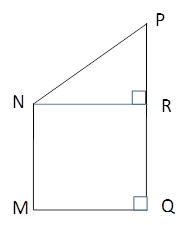Chapter 4.4, Problem 43EElementary Geometry For College St...

7th Edition
Alexander + 2 others
ISBN: 9781337614085

Solutions

Chapter
SectionElementary Geometry For College St...

7th Edition
Alexander + 2 others
ISBN: 9781337614085
Textbook Problem

With M N ¯ | | Q P ¯ and M Q ¯ ⊥ Q P ¯ , MNPQ is a right trapezoid. Finda) m ∠ P , if m ∠ M N P − m ∠ M = 31 ° .b) the length of N R ¯ , if MN = 6 in., NP = 5 in., and QP = 9 in.Exercises 43, 44

To determine

(a)

To Find:

mP

Explanation

Consider the following diagram.

Given: A right trapezoid MNPQ with MN¯||QP¯ and MQ¯QP¯.

Also, mMNPmM=31°.

Here, MN¯||QP¯ and MQ¯QP¯ and mQ=90°.

Then, mM=90°

To determine

(b)

To Find:

The length of NR¯.

Still sussing out bartleby?

Check out a sample textbook solution.

See a sample solution

The Solution to Your Study Problems

Bartleby provides explanations to thousands of textbook problems written by our experts, many with advanced degrees!

Get Started

Place the following set of n = 20 scores in a frequency distribution table.

Essentials of Statistics for The Behavioral Sciences (MindTap Course List)

In Problems 25-28, find the range of the set of numbers given. 25. 3, 5, 7, 8, 2, 11, 6, 5

Mathematical Applications for the Management, Life, and Social Sciences

Proof Prove that limn0101xnyndxdy=0.

Calculus: Early Transcendental Functions (MindTap Course List)

True or false:

Study Guide for Stewart's Multivariable Calculus, 8th

n=12(14)n converges to: a) 94 b) 2 c) 23 d) the series diverges

Study Guide for Stewart's Single Variable Calculus: Early Transcendentals, 8th